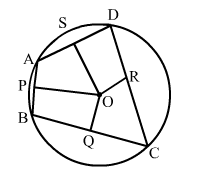# Prove that the perpendicular bisectors of the sides of a cyclic quadrilateral are concurrent.

Question:

Prove that the perpendicular bisectors of the sides of a cyclic quadrilateral are concurrent.

Solution:

Let ABCD be the cyclic quadrilateral and PO, QO, RO and SO be the perpendicular bisectors of sides AB, BC, CD and AD.We know that the perpendicular bisector of a chord passes through the centre of the circle.
Since, AB, BC, CD and AD are the chords of a circle, PO, QO, RO and SO pass through the centre.
i.e., PO, QO, RO and SO are concurrent.
Hence, the perpendicular bisectors of the sides of a cyclic quadrilateral are concurrent.